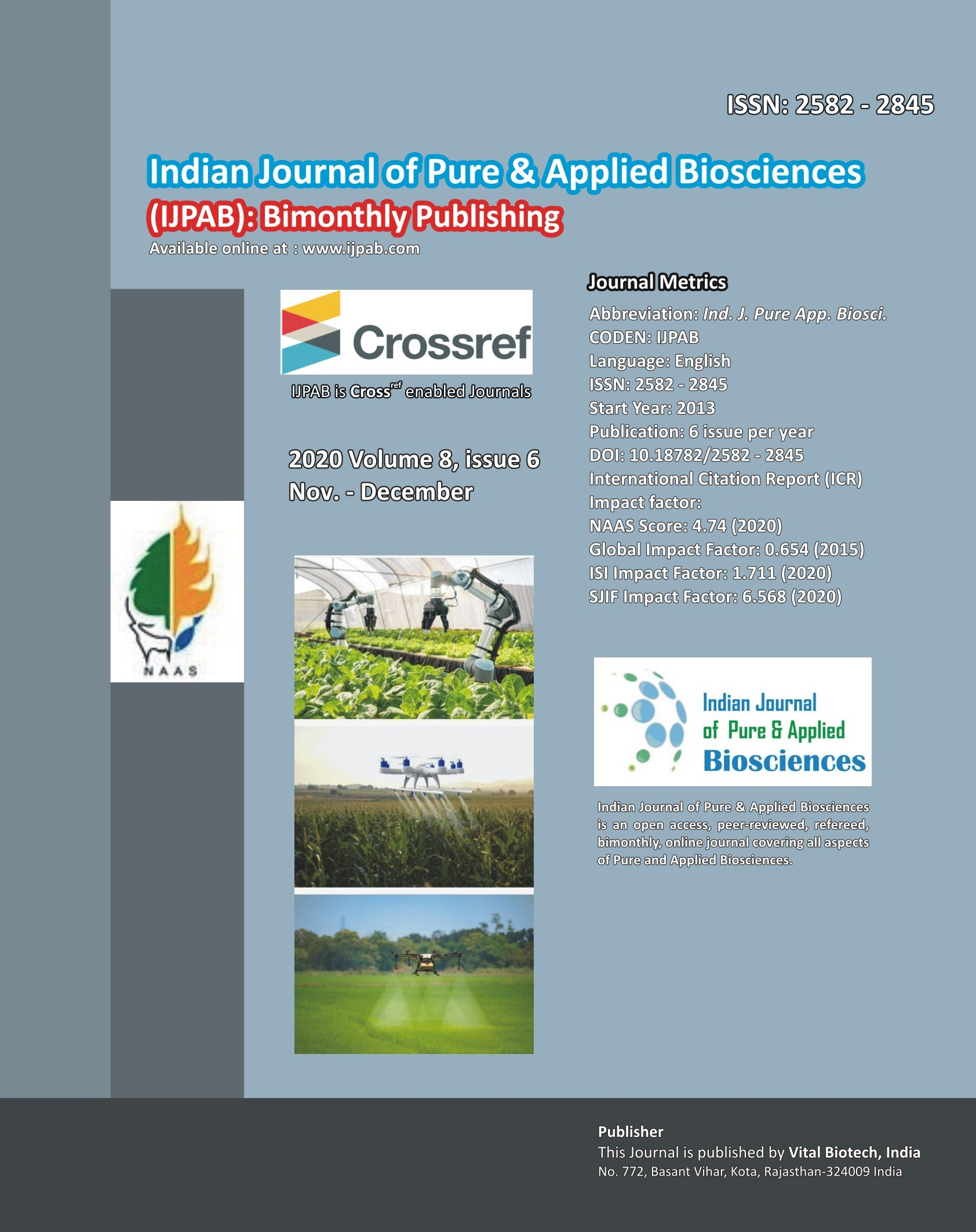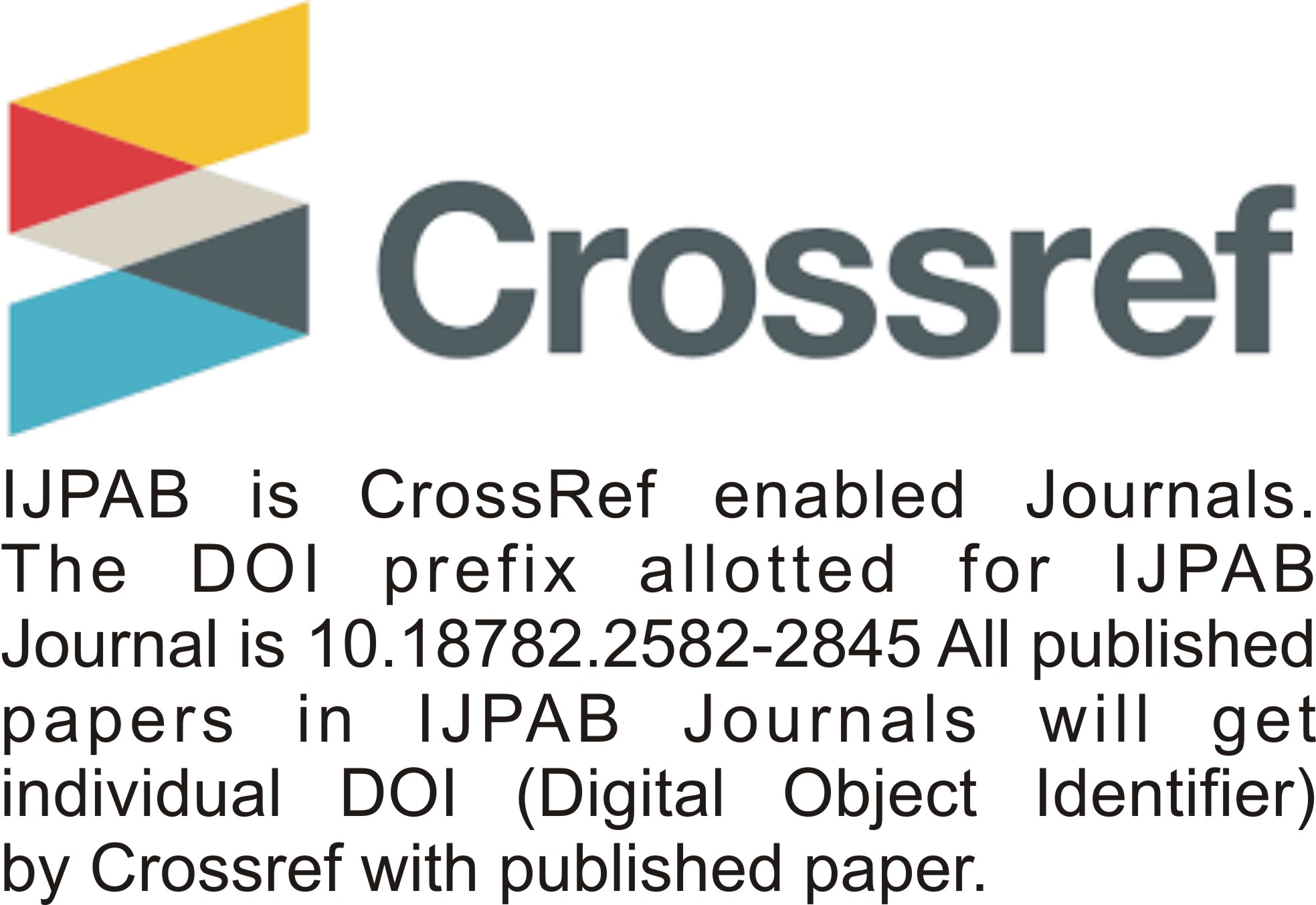INDIAN JOURNAL OF PURE & APPLIED BIOSCIENCES

ISSN (E) : 2582 – 2845

• ###### No. 772, Basant Vihar, Kota

Rajasthan-324009 India

• ###### Call Us On

+91 9784677044

• ###### Mail Us @

editor@ijpab.com

# Archives

Indian Journal of Pure & Applied Biosciences (IJPAB)
Year : 2020, Volume : 8, Issue : 6
First page : (515) Last page : (522)
Article doi: : http://dx.doi.org/10.18782/2582-2845.8487

Improved Linear Regression Using Auxillary Informations

Shabnum Gul*, P.P. Singh and Nisar Ahmad Khan
*Corresponding Author E-mail: khalidzara2018@gmail.com
Received: 15.11.2020 | Revised: 17.12.2020 | Accepted: 26.12.2020

ABSTRACT

The present study was taken under consideration in order to propose improved linear regression using auxiliary information’s of coefficient of regression, coefficient of skewness, coefficient of variation in order to achieve more precision in estimates than the already existing estimators. The properties associated with the proposed estimators are assessed by mean square error and bias and compared with the existing estimators. In the support of the theoretical proposed work we have given numerical illustration.

Keywords: Auxiliary Information, Linear regression, Mean square error, Bias, Efficiency.

Full Text : PDF; Journal doi : http://dx.doi.org/10.18782

Cite this article: Gul, S., Singh, P. P., & Khan, N. A. (2020). Improved Linear Regression Using Auxillary Informations, Ind. J. Pure App. Biosci. 8(6), 515-522. doi: http://dx.doi.org/10.18782/2582-2845.8487

INTRODUCTION

A Regression type estimator using known coefficient of variation is considered and its properties are studied. There are several instances in physical, biological and agricultural sciences where the mean is Proportional to standard deviation and consequently the coefficient of variation is known although the mean and standard deviation may not be known. Some such situations may be seen in Snedecor (1946), Hald (1952), Davies and Goldsmith (1976) and Gleser and Healy (1976). The well-known Weber’s law of psychophysics (see Guilford (1975), Chapter II provides instances where coefficient of variation is known and one such example is given in Singh (1998) also.
Sometimes simple a priori information in the form of coefficient of variation is available to the experimenters in the fields of biology, agriculture, psychophysics etc. Long association of the experimenters with the experimental information concerning the coefficient of variation. This information concerning coefficient of variation. This information concerning coefficient of variation is frequently used to plan experiments, estimate sample size, average, total, etc. (See Searles (1964) also. Further coefficient of variation may be seen in Cochran (1977, 3rd edition) on page 77 and Page 79 of Chapter 4. A good description about knowledge of coefficient of variation is given in Sukhatme et al. (1984) also on page 42.

The objective of the paper is to propose modified estimators for estimating the population mean by using the improved linear regression using auxiliary imformation with the coefficient of regression and coefficient of skewness of the auxiliary variables.

An Illustration
We observe that the conditions discussed in the introduction for known coefficient of variation are satisfied for the data given in Walpoole, R. E., Myres, R. H., Myres, S. L., and Ye, K. (2005, page 473) dealing with measure of aerobic fitness is the oxygen consumption in volume per unit body weight per unit time. Thirty-one individuals were used in an experiment in order to be able to model oxygen consumption (y) against time to run one and half miles (x). consumption of required values has been done and we have the following.

For the population I and II we use the Data Sets data of Singh and Chaudhary (1986) page 177 and for the population III we use the data of Murthy (1967) page 228 in which fixed capital is denoted by X (auxiliary variable) and output of 80 factories are denoted by Y (study variable). For the population IV, the data is of cultivation and production of apple in district Baramulla of Kashmir (Jammu and Kashmir) in which the apple production (in tons) is denoted by Y (study variable) and number of apple trees are denoted by X (auxiliary variable, 1unit = 100 trees) in 117 villages of the Baramulla region of Jammu and Kashmir in 2010-2011 (Source: RC Mproject, pilot survey for estimation of cultivation andproduction of apple in district Baramulla, RC Mapproved project).

Table 1: Characteristics of these populations

 Parameters Population 3 Population 4 80 117 20 15 5182.637 2179 1126.463 560.0 0.941 0.991703 1835.659 862 0.354193 0.9728 845.610 235.5 0.7506772 0.7395 -0.063386 1.10 1.050002 0.20 1795.5 550.5 1040.5 500.6 588.125 200.45 901.081 205.142 801.381 150.600 791.364 98.67 1150.7 594.465

Table 1: Percentage gain in efficiency of the proposed estimator  with respect to  and

 Data set Percentage gain in efficiency  of with respect to Percentage gain in efficiency of with respect to Data 1 260.8 19.6 Data 2 286.78 79.8 Data 3 34.67 67,8

CONCLUSION

The use of proposed estimator is limited for the situations when coefficient of variation is known. However, in case of unknown coefficient of variations its estimated value may be used after studying the performance of the estimator (robustness) against different values of CV, if the guess is in error say 5%, 10%, 15%. 20%, 25%, 50%. Further work is being done in this direction.

REFERENCES

Agrawal, M. C., & Panda, K. B. (1999). A predictive justification for variance estimation using auxiliary information. Jour. Ind. Ag. Statistics, 52(2), 192-200.
Basu, D. (1971). An essay on the logical foundations of statistical inference, Part I, Foundations of Statistical Inference, Ed. By Godambe, V. P. & Sportt, D. A. New York, 203-233.
Cochran, W. G. (1977). Sampling Techniques, 3rdedn. (Wiley & Sons).
Das, A. K. (1988). Contribution to the theory of sampling strategies based on auxiliary information (Ph.D. thesis submitted to Bidhan Chandra Krishi Vishwa vidyalaya, Mohanpur, Nadia, West Bengal, India).
Kadilar, C., & Cingi, H. (2006). Improvement in variance estimation using auxiliary information, Hacettepe Journal of Mathematics and Statistics, 35(1), 111-115.
Panda, K. B., & Sahoo, N. (2015). Systems of exponential ratio-based and exponential product-based estimators with their efficiency. ISOR Journal of Mathematics, 11(3), Ver. I (May-June), PP 73-77.
Panda, K. B., & Das, P. (2018). Efficient hierarchic multivariate product-based estimator. International Journal of Scientific Research in Mathematical and Statistical Sciences, 5(2), pp. 65-69.
Panda, K. B., & Das, P. (2018). Efficient hierarchic predictive multivariate product estimator based on harmonic mean. International Journal of Mathematics Trends and Technoloy (IJMTT) 56(6), pp. 14-18.

Gupta, Sat & Shabbir, Javid (2008). Variance estimation in simple random sampling using auxiliary information. Hacettepe Journal of Mathematics and Statistics, 37(1), 57-67.